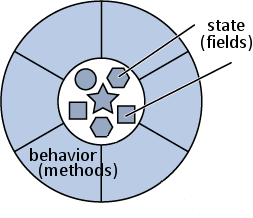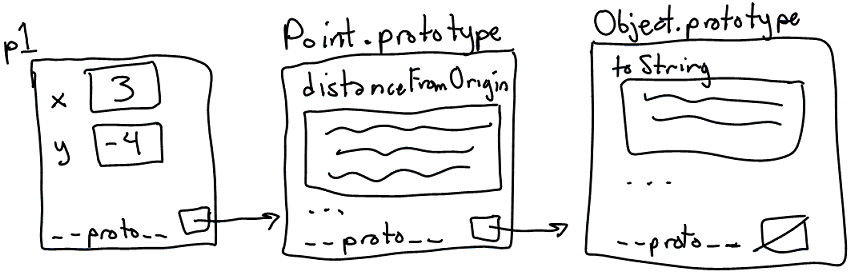# Web Programming Step by Step

### Lecture 27 Object-Oriented JavaScript

Except where otherwise noted, the contents of this presentation are Copyright 2009 Marty Stepp and Jessica Miller.

# Lecture Outline

• Motivation for objects
• Creating custom objects
• Object prototypes and "classes"
• Pseudo-inheritance using prototypes
• The Prototype framework's features for classes and inheritance

# Why use classes and objects?• small programs are easily written without objects
• JavaScript treats functions as first-class citizens
• larger programs become cluttered with disorganized functions
• objects group related data and behavior
• helps manage size and complexity, promotes code reuse
• You have already used many types of JavaScript objects
• Strings, arrays, HTML / XML DOM nodes
• Prototype `Ajax.Request`, Scriptaculous `Effect` / `Sortable` / `Draggable`

# Creating a new anonymous object

```var name = {
fieldName: value,
...
fieldName: value
};
```
```var pt = {
x: 4,
y: 3
};
alert(pt.x + ", " + pt.y);
```
• in JavaScript, you can create a new object without creating a class
• the above is like a `Point` object; it has fields named `x` and `y`
• the object does not belong to any class; it is the only one of its kind
• `typeof(pt) === "object"`

```new Ajax.Request("http://example.com/app.php",
{
method: "get",            // an object with a field named method (String)
onSuccess: ajaxSuccess    // and a method named onSuccess
}
);

{
duration: 2.0,            // an object with 3 fields named:
from: 1.0,                // duration, from, and to (Number)
to: 0.5
}
);
```
• the parameters in `{}` passed to Prototype/Scriptaculous were actually anonymous objects

# Objects that have behavior (functions/methods)

```var name = {
...
methodName: function(parameters) {
statements;
}
};
```
```var pt = {
x: 4,  y: 3,
distanceFromOrigin: function() {
return Math.sqrt(this.x * this.x + this.y * this.y);
}
};

```
• like in Java, objects' methods run "inside" that object
• inside an object's method, the object refers to itself as `this`
• unlike in Java, the `this` keyword is mandatory in JS

# A poor attempt at a "constructor"

What if we want to create an entire new class, not just one object?

• JavaScript, unlike Java, does NOT have classes
• we could emulate the functionality of a constructor with a function:
```// Creates and returns a new Point object. (This is bad code.)
function constructPoint(xValue, yValue) {
var pt = {
x: xValue,  y: yValue,
distanceFromOrigin: function() {
return Math.sqrt(this.x * this.x + this.y * this.y;
}
};
return pt;
}
```
```var p = constructPoint(4, -3);
```
• the above code is ugly and doesn't match the `new` syntax we're used to

# Constructor functions

```// Constructs and returns a new Point object.
function Point(xValue, yValue) {
this.x = xValue;
this.y = yValue;
this.distanceFromOrigin = function() {
return Math.sqrt(this.x * this.x + this.y * this.y);
};
}
```
```var p = new Point(4, -3);
```
• a constructor is just a normal function
• when any function called with `new`, JavaScript does the following:
• creates a new empty anonymous object and uses it as `this` within the function
• implicitly returns the new object at the end of the function
• what happens if our "constructor" is called as a normal function, without `new`?
```var p = Point(4, -3);
```

# Problems with our constructor

```// Constructs and returns a new Point object.
function Point(xValue, yValue) {
this.x = xValue;
this.y = yValue;
this.distanceFromOrigin = function() {
return Math.sqrt(this.x * this.x + this.y * this.y);
};
}
```
• ugly syntax; every method must be declared inside the constructor
• (subtle) replicates the methods in every object (wasteful)
• every `Point` object has its own entire copy of the `distanceFromOrigin` code• prototype: an ancestor of a JavaScript object
• like a "super-object" instead of a superclass
• a parent at the object level rather than at the class level
• not to be confused with Prototype framework
• every object contains a reference to its prototype
• the default is `Object.prototype`
• strings use `String.prototype`, etc.
• a prototype can have a prototype, and so on
• an object "inherits" all methods/data from its prototype(s)
• it doesn't have to make a copy of them, which saves memory
• prototypes allow JavaScript to mimic classes and inheritance

# An object's prototype chain• when you try to look up a property or method in an object, JavaScript:
1. Sees if the object itself contains that property/method.
2. If not, recursively checks the object's prototype to see if it has the property/method.
3. Continues up the "prototype chain" until it finds the property/method or gives up with `undefined`.

# Constructors and prototypes

```// also causes Point.prototype to become defined
function Point(xValue, yValue) {
...
}
```
• every constructor also has an associated prototype object
• example: when we define our `Point` constructor, that creates a `Point.prototype`
• initially this object has nothing in it
• every object you construct will use the constructor's prototype object as its prototype
• example: every constructed `Point` object will use `Point.prototype`
• (revised) when any function called with `new`, JavaScript does the following:
• creates a new empty anonymous object
• attaches the function's prototype object to the new object as its prototype
• runs the constructor's code, using the new object as `this`
• implicitly returns the new object at the end of the function

# Modifying a prototype

```// adding a method to the prototype
className.prototype.methodName = function(parameters) {
statements;
}
```
```Point.prototype.distanceFromOrigin = function() {
return Math.sqrt(this.x * this.x + this.y * this.y);
};
```
• adding a method/field to a prototype will give it to all objects using that prototype
• better than manually adding each method to each object (copying the method N times)
• we generally put only methods and constant data (not fields!) in a prototype object
• what would happen if we put the `x` and `y` fields in `Point.prototype`?
• Exercise: Add `distance` and `toString` methods.

# `Point` prototype methods

```// Computes the distance between this point and the given point p.
Point.prototype.distance = function(p) {
var dx = this.x - p.x;
var dy = this.y - p.y;
return Math.sqrt(dx * dx + dy * dy);
};

// Returns a text representation of this object, such as "(3, -4)".
Point.prototype.toString = function() {
return "(" + this.x + ", " + this.y + ")";
};
```
• our `Point` code could be saved into a file `Point.js`
• the `toString` method works similarly as in Java

# Modifying built-in prototypes

```// add a 'contains' method to all String objects
String.prototype.contains = function(text) {
return this.indexOf(text) >= 0;
};

// add a 'lightUp' method to all HTML DOM element objects
HTMLElement.prototype.lightUp = function() {
this.style.backgroundColor = "yellow";
this.style.fontWeight = "bold";
};
```
• ANY prototype can be modified, including those of existing types
• Prototype and other libraries do this
• not quite the same as adding something to a single object
• Exercise: Add a `reverse` method to strings.
• Exercise: Add a `shuffle` method to arrays.

# Practice problem: Favorite Movies• Add JavaScript to `moviegallery.html` to list your favorite movies.
• Create a new type called `Movie`:
• `Movie(title, genre, rating)` - constructor.
• `createHTML()` - Creates and returns an HTML DOM object representing a `div` holding the HTML for this movie.
```<div class="movie">
<button class="delete">X</button>
<p class="title">Indiana Jones 4</p>
<p class="rating">3/5</p>
</div>
```
• `deleteMe()` - Removes the movie from the page.

# Pseudo-inheritance with prototypes

```function SuperClassName(parameters) {   // "superclass" constructor
...
};
```
```function SubClassName(parameters) {     // "subclass" constructor
...
};
```
```SubClassName.prototype = new SuperClassName(parameters);   // connect them
```

• to make a "subclass", tell its constructor to use a "superclass" object as its prototype
• why not just write it this way?
```SubClassName.prototype = SuperClassName.prototype;   // connect them
```

# Pseudo-inheritance example

```// Constructor for Point3D "class"
function Point3D(x, y, z) {
this.x = x;
this.y = y;
this.z = z;
};

Point3D.prototype = new Point(0, 0);   // set as "subclass" of Point

// override distanceFromOrigin method
Point3D.prototype.distanceFromOrigin = function() {
return Math.sqrt(this.x * this.x + this.y * this.y + this.z * this.z);
};
```
• mostly works fine, but there no equivalent of the `super` keyword
• no built-in way to call an overridden method
• no easy way to call the superclass's constructor

# Classes and prototypes

• limitations of prototype-based code:
• unfamiliar / confusing to many programmers
• somewhat unpleasant syntax
• difficult to get inheritance-like semantics (subclassing, overriding methods)

# Creating a class

```className = Class.create({
// constructor
initialize : function(parameters) {
this.fieldName = value;
...
},

methodName : function(parameters) {
statements;
},
...
});
```
• constructor is written as a special `initialize` function

# `Class.create` example

```Point = Class.create({
// Constructs a new Point object at the given initial coordinates.
initialize: function(initialX, initialY) {
this.x = initialX;
this.y = initialY;
},

// Computes the distance between this Point and the given Point p.
distance: function(p) {
var dx = this.x - p.x;
var dy = this.y - p.y;
return Math.sqrt(dx * dx + dy * dy);
},

// Returns a text representation of this Point object.
toString: function() {
return "(" + this.x + ", " + this.y + ")";
}
});
```

# Inheritance

```className = Class.create(superclass, {
...
});
```
```// Points that use "Manhattan" (non-diagonal) distances.
ManhattanPoint = Class.create(Point, {
// Computes the Manhattan distance between this Point and p.
// Overrides the distance method from Point.
distance: function(p) {
var dx = Math.abs(this.x - p.x);
var dy = Math.abs(this.y - p.y);
return dx + dy;
},

// Computes this point's Manhattan Distance from the origin.
distanceFromOrigin: function() {
return this.x + this.y;
}
});
```

# Referring to superclass: `\$super`

```name: function(\$super, parameters) {
statements;
}
```
```ManhattanPoint3D = Class.create(ManhattanPoint, {
initialize: function(\$super, initialX, initialY, initialZ) {
\$super(initialX, initialY);   // call Point constructor
this.z = initialZ;
},

// Returns 3D "Manhattan Distance" from p.
distance: function(\$super, p) {
var dz = Math.abs(this.z - p.z);
return \$super(p) + dz;
},
});
```
• can refer to superclass's overridden method as `\$super` in code

# Practice problem: Fancy movies• Modify the movie gallery code from the last practice problem so that it uses Prototype's `Class.create` method to make the `Movie` type.
• Once this is done, add a new subtype `FancyMovie` that displays its movie ratings as star images rather than just showing a number on the page.